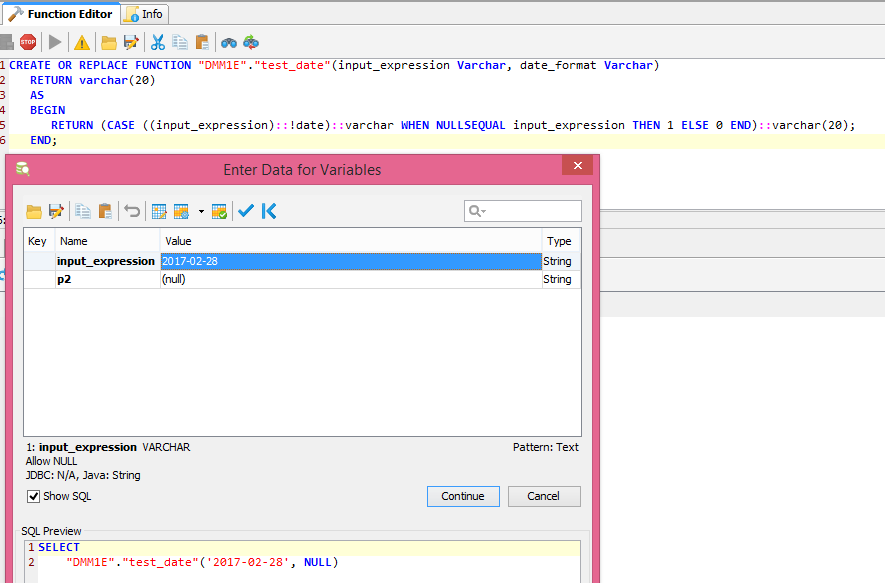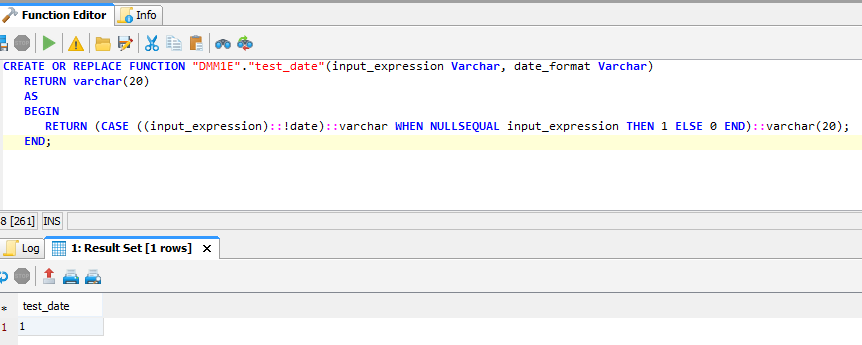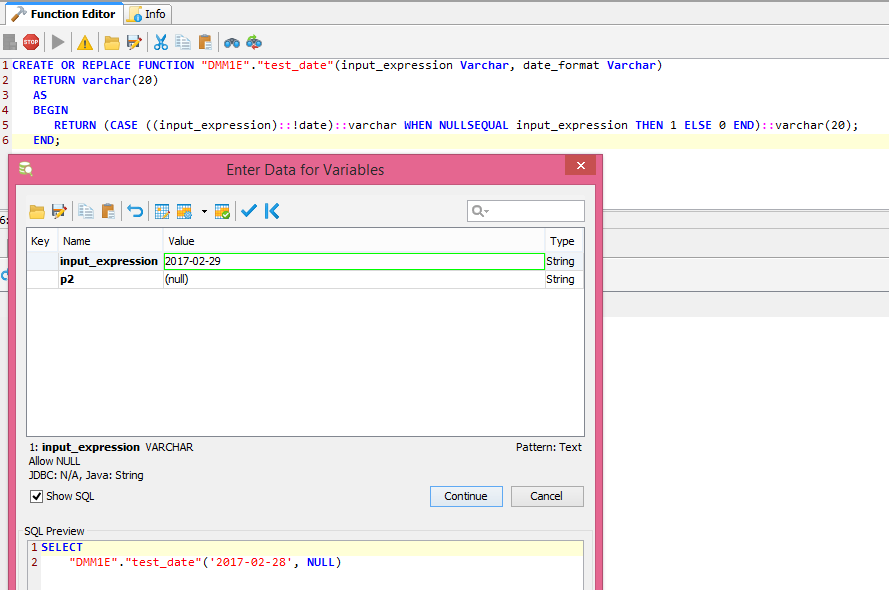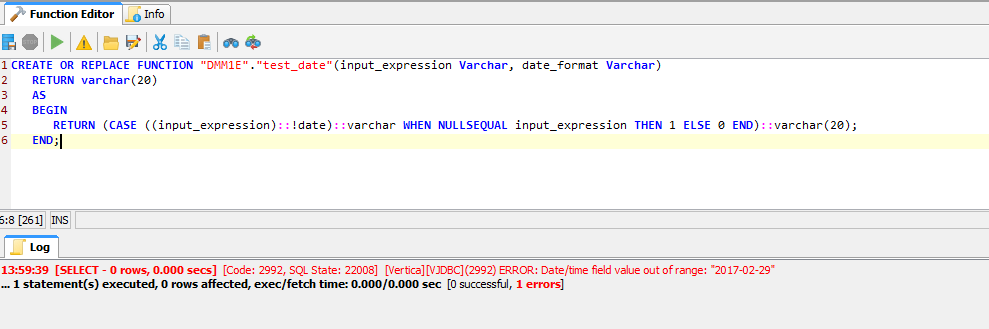# "isdecimal(input_expression, decimal_format)" function in vertica

Hi support,

Is the "Isdecimal" function avaible under vertica. It's the same question for isdate() and isint()

Thanks

• You can create your own SQL functions....

Simple Example:

```dbadmin=> create function isdecimal(x varchar) return varchar
CREATE FUNCTION

isdecimal
-----------
1
(1 row)

isdecimal
-----------
1
(1 row)

isdecimal
-----------
0
(1 row)

isdecimal
-----------
1
(1 row)
```
• Hi support,

I tested your SQL function and it works for positive numbers, by changing the REGEXP_COUNT it works also for negative numbers. However, I wanted to have this possibility to pass in parameter the format of the expected decimal. Can you explain to me how to implement this in vertica ? I want a SQL function that looks like this below.
isdecimal(input_expression, decimal_format)
Examples:
isdecimal('-10,905', '- #.##') ==> return 0 because it does not match the expected format
isdecimal('-10,905', '#.##') ==> return 0 because it does not match the expected format
isdecimal('-10,905', '- #.###') ==> return 1 because it matches the expected format
isdecima(‘2’) ==> return 1

etc…

• - Select Field - Administrator
edited November 2017

I'm sure there are a million ways to create the function you want! Here's one way to get you started:

```create or replace function isdecimal(x varchar, y varchar) return boolean
as
begin
return REGEXP_COUNT(x, '\.') = 1 and
LENGTH(split_part(x, '.', 2)) = LENGTH(split_part(y, '.', 2)) and
REGEXP_COUNT(split_part(x, '.', 1), '^(-?[1-9]+\d*)\$|^0\$') and
REGEXP_COUNT(split_part(x, '.', 2), '^([1-9]+\d*)\$|^0\$');
end;
```

Here is the output of your samples:

```dbadmin=> select isdecimal('-10.905', '-#.##');
isdecimal
-----------
f
(1 row)

isdecimal
-----------
f
(1 row)

isdecimal
-----------
t
(1 row)

ERROR 3457:  Function isdecimal(unknown) does not exist, or permission is denied for isdecimal(unknown)
HINT:  No function matches the given name and argument types. You may need to add explicit type casts
```

For that last one, it failed 'cause no format string was passed. But is 2 a decimal? I think 2.0 is.

```dbadmin=> select isdecimal('2.0', '#.#');
isdecimal
-----------
t
(1 row)
```

Anyway, you can modify the code to handle what ever case you want and also check the format string, i.e. does it only contain a negative sign and # characters?

You can also go back to the original REGEX, I was just trying out the SPLIT_PART function...

```dbadmin=> create or replace function isdecimal(x varchar, y varchar) return boolean
dbadmin->   return REGEXP_COUNT(x, '^(-?\d*\.)?\d+\$') and LENGTH(split_part(x, '.', 2)) = LENGTH(split_part(y, '.', 2));
```
• Hi,

After several tests this method does not cover all cases.
I wanted to proceed as follows:

CREATE OR REPLACE FUNCTION "DMM1E"."test_date"(input_expression Varchar, date_format Varchar)
RETURN varchar(20)
AS
BEGIN
RETURN (CASE ((input_expression)::!date)::varchar WHEN NULLSEQUAL input_expression THEN 1 ELSE 0 END)::varchar(20);
END;

I wanted to store the result (input_expression) ::! date in a variable you can tell me how to use a variable in vertica function(SQL).
When I call the function with test_date ('2017-02-29',) I get the error message below. I want to get 0. Thank you for your help.

... Physical database connection acquired for:
13:47:55 [SELECT - 0 rows, 0.016 secs] [Code: 2992, SQL State: 22008] [Vertica]VJDBC ERROR: Date/time field value out of range: "2017-02-29"... 1 statement(s) executed, 0 rows affected, exec/fetch time: 0.016/0.000 sec [0 successful, 1 errors]• - Select Field - Administrator
edited November 2017

Hi,

User Defined SQL Functions have to be pretty simple. There is no way to store data in variables or handle the type of error you are receiving.

Maybe the following function that uses yet another REGEXP can help?

Note: The regular expression matches ANSI SQL date format YYYY-mm-dd hh:mi:ss am/pm. You can use / - or space for date delimiters, so 2017-12-31 works just as well as 2017/12/31. It also checks leap year from 1901 to 2099.

```dbadmin=> create or replace function isdate(x varchar) return varchar
CREATE FUNCTION

isdate
--------
1
(1 row)

isdate
--------
0
(1 row)